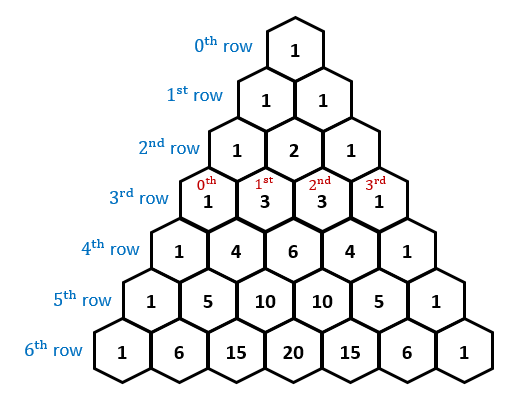# 1.1 Arithmetic sequences and series

#### Sequence

Set of quantities arranged in a definite order, for instance 1, 2, 3, 4... or -1, 2, -4, 8...

• un - nth term, u1 - first term, u2 - second term...

Series

Result of the addition of the terms of a sequence.

• Sigma notation (∑): The sum of...

#### Arithmetic

• Common difference (d): Difference (d) between consecutive terms is always the same.

• d = un - un-1

• Sequence:​ un = a + (n - 1)d; a = u1first term

• Series: Sum of the n terms = Sn = n/2 [2a + (n-1)d] = n/2 (u1 + un)

#### Geometric

• Common ratio (r): Common ratio (r) between consecutive terms is always the same.

• r = un/un-1

• Sequence:​ un = a x r^n-1

• Series: Sum of the n terms = Sn = a (1 - r^n)/(1 - r), │r│< 1 or Sn = a (r^n - 1)/(r -1), r│> 1

• Convergent series: ​Geometric series with │r│< 1 (terms get smaller as n increases)

• Sum of all sequence's terms will tend to approach a certain value: ​S= a/(r -1)

#### Applications

• Compound interest: \$ invested at r % p. a. (per annum) compound interest

• An = P (1 + r/100)^n, where An is the amount after n time periods

• Superannuation: Investing each year the same amount in a scheme

• Total money at the end of the scheme is the sum of each investment done each year

• Sna (r^n - 1)/(r -1) = P (1 + r/100)[1 - (r/100)^n]/[1 - (1 + r/100)]; P = ​initial investment

# 1.2 Exponents and logarithms

#### Base e

Euler's number (e): An irrational number (like ) found in natural patterns.

Exponential function

#### f(x) = a^x, x ϵ R, a > 0 and a ≠ 1

• Graphs with a > 1 (y-values increase as x-values increase)

• Called "exponential growth"

• Cases: When x < 0 then 0 < y < 1; When x>0 then y > 1; At x = 0, y = 1

• Horizontal asymptote at y = 0

• Graphs with 0 < a < 1 ​(y-values decrease as x-values increase)

• Called "exponential decay"

• f(x) = a^-x = (1/a)^x

• Hori​zontal translation: y = a^(x +- k); Vertical translation: y = a^x +- k; Dilation along y-axis: y = ka^x

Logarithms

y = b^N means that N = logb(y), y ≥ 0 and b ≠ 1 and loge(x) = ln(x)

Logarithm rules#### Reflection of the exponential upon the line y = x

• Horizontal translation: y  = log a (x +- k)

• +k: Assymptote ​at -k

• -k: Assymptote at +k

• Vertical translation: y  = log a (x) +- k

• Stretching and shrinking along the y-axis: y  = k log a (x)

• If k<0: Reflection about x-axis​

• Reflection about y-axis: ​y  = log a (-x)

#### Examples (not IB!)

• Richter scale: Magnitude of an earthquake

• R(magnitude) = log 10 (I/Io)

• Ehrenberg relation: Measurement of a children's weight​.

# 1.3 Counting principles and binomial theorem

#### Counting principles

Permutation (Arrangement): Counting process where the order must be taken into account (AC ≠ CA).

• Rule 1: If any one of n different mutually exclusive and exhaustive events can occur on each of k trials, the number of possible outcomes is equal to n^k.

• Example: Dice rolled twice 6^2 = 36 events.

• Rule 2: If there are n1 events on the first trial, n2 events on the second trial and so on, and finally, nk on the kth trial, then the number of possible outcomes is equal to nnx ... x nk.

• Example: Three different pairs of pants, four different shirts and five different shirts 3x4x5 = 60 ways to dress

• Rule 3: The total number of ways that n different objects can be arranged in order is equal to                  n! (factorial) = n x (n-1) x (n-2) x ... x 3 x 2 x 1 (0! = 1)

• Example: In how many ways can seven children sit on a park bench? 7! = 7 x 6 x 5 x 4 x 3 x 2 x 1 = 5040 arrangements.

• Rule 4: The total number of ways of arranging n objects, taking r at a time is given by nPr = n!/(n-r)!

• Example: Total number of arrangements of 8 books on a bookshelf if only 5 are used is given  by 8P5 = 8!/(8-5)!

• Box Method: Filling available spaces, represented by boxes.

• Rule 5: The number of permutations of n objects of which n1 are identical, n2 are identical, ..., nk are identical is given by n!/(n1! x n2! x ... x nk!)

• Useful for anagrams with words.​

Combination (Selection): Counting process where the order has no importance (AC = CA).

• Rule 6: The total number of ways of selecting n objects, taking r at a time, for instance, a selection of 5 books from 8 different books, is given by:#### Binomial theorem

Binomials: Expressions made out of 2 terms (bi), e.g. (a + b)^2

• Powers of aStart at n and decrease a^n, a^(n-1), a^(n-2), a^(n-3), ..., a^0

• Powers of bStart at 0 and increase: b^0, b^1, b^2, b^3, ..., b^n

• Coefficients follow Pascal's triangle: Numbers in the body are found by adding the two numbers immediately above to either side• Example: (x +4)^4 = 1x^4 + 4(x^3)a +6(x^2)(a^2) + 4(x)(a^3) + 1a^6

• 1 4 6 4 1 corresponds to the 4th row in the Pascal's triangle​.

• If there is a minus sign (-), it alternates between the coefficients: + ... - ... + ... - ...​

The general term: (x + a)^n = (